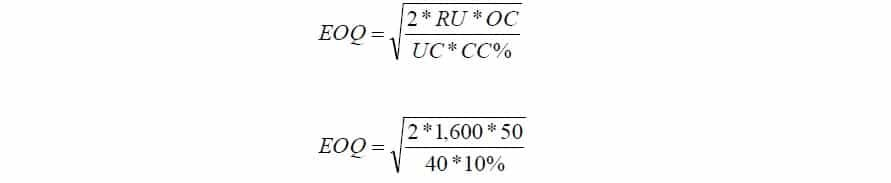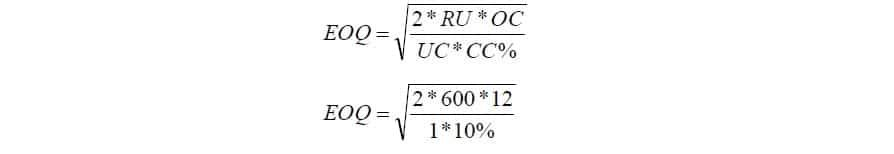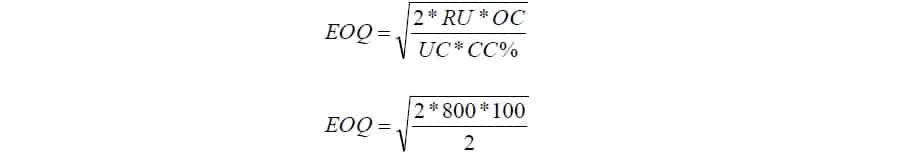# Economic Order Quantity Problems and Solutions

Economic Order Quantity Unsolved Problem PDF

## Problem # 1:

Calculate Economic Order Quantity (EOQ) from the following:

Annual consumption           6,000 units

Cost of ordering                 Rs. 60

Carrying costs                    Rs. 2

### Solution:EOQ =600 Units

## Problem # 2:

From the following particulars, calculate the Economic Order Quantity (EOQ):

Annual requirements          1,600 units                          Cost of materials per units                      Rs. 40

Cost of placing and receiving one order:      Rs. 50       Annual carrying cost for inventory value       10%

### Solution:EOQ = 200 Units

## Problem # 3:

Calculate EOQ from the following?

Consumption during the year = 600 units              Ordering cost Rs. 12 per order

Carrying cost 20%                                                  Selling Price per unit Rs. 20

### Solution:Economic Order Quantity = 379 Units

>> Practice Inventory Management Problems and Solutions.

## Problem # 4:

A manufacturer buys certain equipment form suppliers at Rs. 30 per unit. Total annual needs are 800 units. The following further data are available:

Annual return on investments 10%                    Rent, insurance, storing per unit per year Rs. 2

Cost of placing an order Rs. 100

Required: EOQ

### Solution:EOQ = 200 Units

## Problem # 5:

From the figures given below, calculate Economic Order Quantity (EOQ) and Total cost at EOQ?

Total consumption of material per year         10,000 kgs                Buying cost per order Rs. 50

Unit cost of material                                        Rs. 2 per kg            Carrying and storage cost 8%

### Solution:EOQ = 2,500 Units

Total Inventory Cost = [Fixed ordering cost (F) * Number of Order per year N] + [Carrying Cost (C)* EOQ/2]

Total Inventory Cost = [50 * 10,000/2,500] + [(2*0.08)* 2,500/2]

Total Inventory Cost = 200 + 200

Total Inventory Cost = Rs.  400

### Related Topics

Inventory Management

Inventory Management MCQs

Inventory Management Problems

Economic Order Quantity MCQ

Economic Order Quantity

### More Interest

Labor Cost

Payroll Systems

Labor Cost MCQs

Labor Cost Problems

Inventory Valuation

1. A manufacturer uses 7500 units of a material ‘Z’ per year. The material cost is rs.15 per unit and carrying cost is 40% per annum of average inventory cost. The cost of placing order is rs.36. Calculate EOQ and number of orders placed per annum.

2. Help me with this problem
Data given.
EOQ=750 units.
Annual demand= 22500units.
Carrying cost= 20% of average stock value.
Requirements;
a. Unit purchase price
b. Ordering cost per order.
c. Number of order per annum.
d. Annual ordering cost.

• I need the solution of this question

• it is not explicit question please do it?

A particular item has a demand of 9000 units per year. The cost of one procurement is N100 and the holding cost per unit is ₦2.40 per year. The replacement is instantaneous and no shortages are allowed.
Determine:
(i) The number of orders per year
(ii) The time between orders
(iii) The total cost per year if the cost of one unit is ₦10

4. a close manufacture purchase cotten oin large quantity from producers in order to make different types of close. 250000 tones of cotton are forecasted to be required next year to support the production of the out put. if cotton cost birr 600 per ton carring cost is 20% of the purchasing cost and ordering cost birr 1500 per order the farm operators 240 days per year and its daily requirement is 500 tons of cost. how many time per year should the firm reorder

• The question has no lead time.

5. DVD Store sells rice per cavan in some small stores. The current price schedule given to small stores is as follows:
5-15 cavans, ₱1,200 per cavan
16-30 cavans, ₱1,100 per cavan
More than 30 cavans, ₱1,000 per cavan
Average annual demand is 1000 cavans of rice. Carrying cost is ₱650 per cavan per year. Order cost is ₱350. Determine the optimal order quantity.

6. Why

7. the xyz import company imports olives as well as other items used by specialty restaurants. the company has determined that the ordering cost of an order of extra fenly olives is \$ 90 and the carrying charges are 40% of the average value of the inventory. xyz buys approximately \$ 1350000 of the olives annually. currently the company is importing the olives on an optimal eoq basis but has been given the option of purchasing the olives for 50% discount if purchases are made exactly six times a year. Should the company keep this arrangement?

• problem solving quesion

8. The Florida company has obtained the following costs and other data pertaining to one of its materials:
Workign days per year 250
Normal use per day 500 units
Max. use per day 600 units
Min. use per day 100 units
variable cost of placing one order \$ 36
variable carrying cost per unit per year \$ 1

Required:

Economic Order Quantity

9. Hi,
I have calculated the EOQ . Now i want to order the items on 2 week basis.
How can I redefine the formula.

10. What do we mean when we talk about Normal usage in units per year? is it the same as consumption?

• Can you work out for me this problem?

Normal delivery time: 2.5 weeks
Maximum delivery time: 3.5 weeks
Normal usage: 52 000 units per year
Purchase price per unit: N\$8.50
Cost of placing an order: N\$18.00
Interest rate: 2% per year
Storing cost per unit: N\$2.50

Requirement
2.2.1 Calculate the Economic Order Quantity (EOQ). 
2.2.2 Calculate the re-order point if the organisation does not keep safety (minimum level) inventory. 
2.2.3 Calculate the re-order point if the organisation has a policy to keep safety inventory. 
2.2.4 Calculate the safety inventory that should be kept by the organisation. 
2.2.5 Using your answers to the calculations above where relevant, calculate the company’s total inventory costs (holding plus ordering) for the year. 

Thank you

11. I am no longer certain the place you are getting your information, however good topic.
I must spend a while studying much more or figuring out more.

12. I like the helpful information you provide in Economic Order Quantity Problems and Solutions. I will bookmark your blog and check again here frequently. I am quite sure I will learn plenty of new stuff right here! Good luck for the next!

13. Hi! I’ve been following your blog for a long time now and finally got the bravery to go ahead and give you a shout out from Dallas Texas! Just wanted to mention keep up the good job!|

• A manufacturer requires 4,000kg. of a raw material
annually. The ordering cost is Rs. 5 per order. The
carrying cost is estimated to be 8% of average inventory
per year. The purchase price of the raw material is Rs. 2
per kg. Calculate the Economic lot size and the total cost.

14. I visited multiple web sites but the audio feature for audio songs present at this web page is in fact marvelous.|

15. it’s really helpful for us, keep it up !!!!!!!

I also didn’t understand Q3 Holding cost?

• I suppose question 3 EOQ should have been 60 units

16. Quality articles is the secret to interest the visitors to visit the site, that’s what this web page is providing.

• A manufacturer requires 4,000kg. of a raw material
annually. The ordering cost is Rs. 5 per order. The
carrying cost is estimated to be 8% of average inventory
per year. The purchase price of the raw material is Rs. 2
per kg. Calculate the Economic lot size and the total cost.
The manufacturer is offered a 5% discount in purchase.

18. I didn’t understand problem 3 where UC =1 and CC=10%????